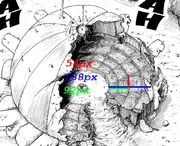## FANDOM

25,784 Pages

Credit goes to Therefir for doing the original calc here. I'll explain the issues with this one as we proceed with the calc.

## First part: The figure

As we can see in this image, the figure isn't a rectangular tank in its entirety. A stadium isn't built up like one, and two figures come out to find the volume, where the outer side of the stadium IS a rectangular tank, but the inner part (which the original calc takes into account) it's actually a triangular prism.

Note: The figure is actually a lot more complex with curvatures and stuff, but Bambu told me that the only way this could work while accounting everything would be with a model program and I don't have the knowledge nor the material to work with one.

## Second part: The dimensions

I'll go with Therefir's values because I find them actually accurate, so the stadium's heigh is 22.75m until the upper half of the seats, where the hole ends going upside. The second picture shows even the fourth stage is destroyed, but that could've been Hiei's do before finishing the fight.Stadium's height = 54px = 22.75m

Stadium's total width = 188px = 79.2m.

Stadium's inner half width = 92px = 38.76m.

## Third part: The volumes

### Triangular prism

Like I said before, the first figure is a triangular prism. The stage isn't even in the closests sections after all.

Volume = 1/2*length*width*height

1/2*38.76*17.37*22.75 = 7658.34615m^3 or 7658346150cm^3

### Rectangular Tank

1/2*40.44*22.75*17.37

Put these values here and the result is 15980.5737m^3 or 15980573700cm^3.

## Calculating the energy

Total volume = 23638919850cm^3

Value used for vaporization of steel = 60117.42j/cc

23638919850cm^3 * 60117.42j/cc = 1421110872968787 joules or 339.65 Kilotons. Large Town level. So about 15x higher than the previous result.

The discussion will come later. I want this to be evaluated first.

Community content is available under CC-BY-SA unless otherwise noted.